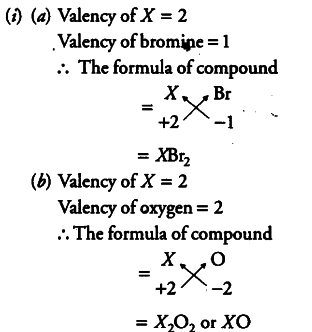# Define formula unit mass of a substance

(i) An element X has a valency of 2. Write the chemical formula for
(a) bromide of the element
(b) oxide of the element.
(ii) Define formula unit mass of a substance.(ii) Formula unit mass is the sum of atomic masses of all atoms present in a formula unit of compound.